# HashMap多线程并发问题分析

## 并发问题的症状

#### 多线程put后可能导致get死循环

package com.king.hashmap;

import java.util.HashMap;

public class TestLock {

private HashMap map = new HashMap();

public TestLock() {
public void run() {
for (int i = 0; i < 50000; i++) {
map.put(new Integer(i), i);
}
System.out.println("t1 over");
}
};

public void run() {
for (int i = 0; i < 50000; i++) {
map.put(new Integer(i), i);
}

System.out.println("t2 over");
}
};

public void run() {
for (int i = 0; i < 50000; i++) {
map.put(new Integer(i), i);
}

System.out.println("t3 over");
}
};

public void run() {
for (int i = 0; i < 50000; i++) {
map.put(new Integer(i), i);
}

System.out.println("t4 over");
}
};

public void run() {
for (int i = 0; i < 50000; i++) {
map.put(new Integer(i), i);
}

System.out.println("t5 over");
}
};

public void run() {
for (int i = 0; i < 50000; i++) {
map.get(new Integer(i));
}

System.out.println("t6 over");
}
};

public void run() {
for (int i = 0; i < 50000; i++) {
map.get(new Integer(i));
}

System.out.println("t7 over");
}
};

public void run() {
for (int i = 0; i < 50000; i++) {
map.get(new Integer(i));
}

System.out.println("t8 over");
}
};

public void run() {
for (int i = 0; i < 50000; i++) {
map.get(new Integer(i));
}

System.out.println("t9 over");
}
};

public void run() {
for (int i = 0; i < 50000; i++) {
map.get(new Integer(i));
}

System.out.println("t10 over");
}
};

t1.start();
t2.start();
t3.start();
t4.start();
t5.start();

t6.start();
t7.start();
t8.start();
t9.start();
t10.start();
}

public static void main(String[] args) {
new TestLock();
}
}


CPU利用率过高一般是因为出现了出现了死循环，导致部分线程一直运行，占用cpu时间。问题原因就是HashMap是非线程安全的，多个线程put的时候造成了某个key值Entry key List的死循环，问题就这么产生了。

at java.util.HashMap.get(HashMap.java:303)
at com.sohu.twap.service.logic.TransformTweeter.doTransformTweetT5(TransformTweeter.java:183)

at java.util.HashMap.put(HashMap.java:374)
at com.sohu.twap.service.logic.TransformTweeter.transformT5(TransformTweeter.java:816)

### put非null元素后get出来的却是null

void transfer(Entry[] newTable) {
Entry[] src = table;
int newCapacity = newTable.length;
for (int j = 0; j < src.length; j++) {
Entry e = src[j];
if (e != null) {
src[j] = null;
do {
Entry next = e.next;
int i = indexFor(e.hash, newCapacity);
e.next = newTable[i];
newTable[i] = e;
e = next;
} while (e != null);
}
}
}


if (e != null) {
src[j] = null;


## HashMap数据结构

HashMap通常会用一个指针数组（假设为table[]）来做分散所有的key，当一个key被加入时，会通过Hash算法通过key算出这个数组的下标i，然后就把这个 插到table[i]中，如果有两个不同的key被算在了同一个i，那么就叫冲突，又叫碰撞，这样会在table[i]上形成一个链表。

## HashMap的rehash源代码

public V put(K key, V value)
{
......
//算Hash值
int hash = hash(key.hashCode());
int i = indexFor(hash, table.length);
//如果该key已被插入，则替换掉旧的value （链接操作）
for (Entry<K,V> e = table[i]; e != null; e = e.next) {
Object k;
if (e.hash == hash && ((k = e.key) == key || key.equals(k))) {
V oldValue = e.value;
e.value = value;
e.recordAccess(this);
return oldValue;
}
}
modCount++;
//该key不存在，需要增加一个结点
addEntry(hash, key, value, i);
return null;
}


void addEntry(int hash, K key, V value, int bucketIndex)
{
Entry<K,V> e = table[bucketIndex];
table[bucketIndex] = new Entry<K,V>(hash, key, value, e);
//查看当前的size是否超过了我们设定的阈值threshold，如果超过，需要resize
if (size++ >= threshold)
resize(2 * table.length);
}


void resize(int newCapacity)
{
Entry[] oldTable = table;
int oldCapacity = oldTable.length;
......
//创建一个新的Hash Table
Entry[] newTable = new Entry[newCapacity];
//将Old Hash Table上的数据迁移到New Hash Table上
transfer(newTable);
table = newTable;
threshold = (int)(newCapacity * loadFactor);
}


void transfer(Entry[] newTable)
{
Entry[] src = table;
int newCapacity = newTable.length;
//下面这段代码的意思是：
//  从OldTable里摘一个元素出来，然后放到NewTable中
for (int j = 0; j < src.length; j++) {
Entry<K,V> e = src[j];
if (e != null) {
src[j] = null;
do {
Entry<K,V> next = e.next;
int i = indexFor(e.hash, newCapacity);
e.next = newTable[i];
newTable[i] = e;
e = next;
} while (e != null);
}
}
}


## 正常的ReHash过程

1. 我假设了我们的hash算法就是简单的用key mod 一下表的大小（也就是数组的长度）。
2. 最上面的是old hash 表，其中的Hash表的size=2, 所以key = 3, 7, 5，在mod 2以后都冲突在table1这里了。
3. 接下来的三个步骤是Hash表 resize成4，然后所有的 重新rehash的过程。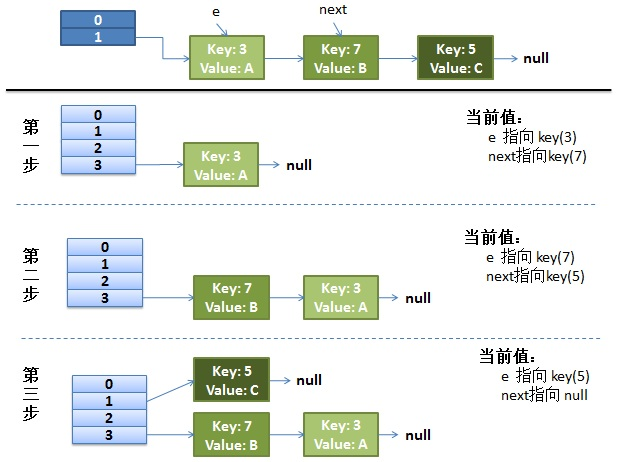## 并发的Rehash过程

#### （1）假设我们有两个线程。我用红色和浅蓝色标注了一下。我们再回头看一下我们的 transfer代码中的这个细节：

do {
Entry<K,V> next = e.next; // <--假设线程一执行到这里就被调度挂起了
int i = indexFor(e.hash, newCapacity);
e.next = newTable[i];
newTable[i] = e;
e = next;
} while (e != null);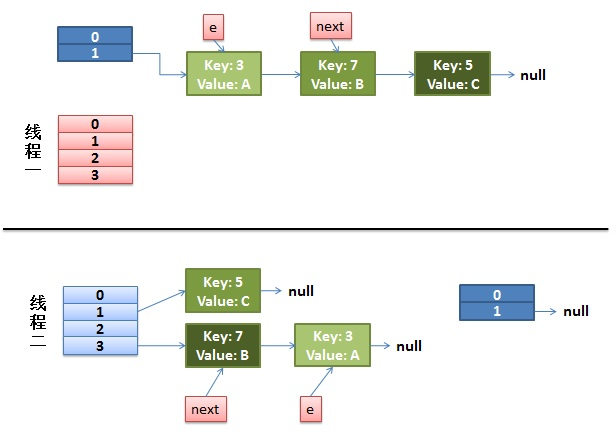#### （2）线程一被调度回来执行。

1. 先是执行 newTalbe[i] = e。
2. 然后是e = next，导致了e指向了key(7)。
3. 而下一次循环的next = e.next导致了next指向了key(3)。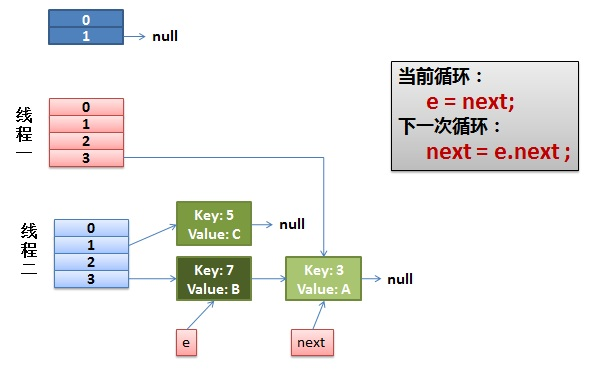#### （3）一切安好。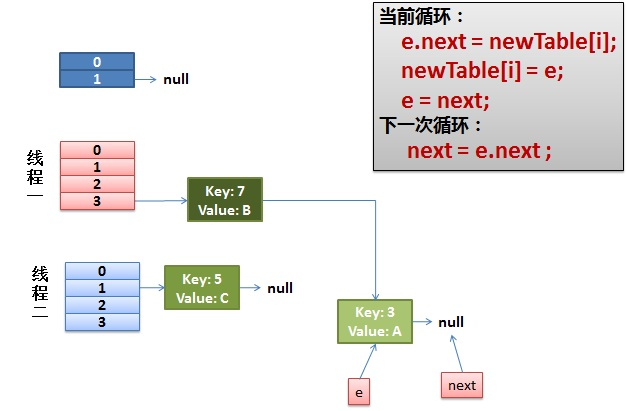#### （4）环形链接出现。

e.next = newTable[i] 导致 key(3).next 指向了 key(7)。注意：此时的key(7).next 已经指向了key(3)， 环形链表就这样出现了。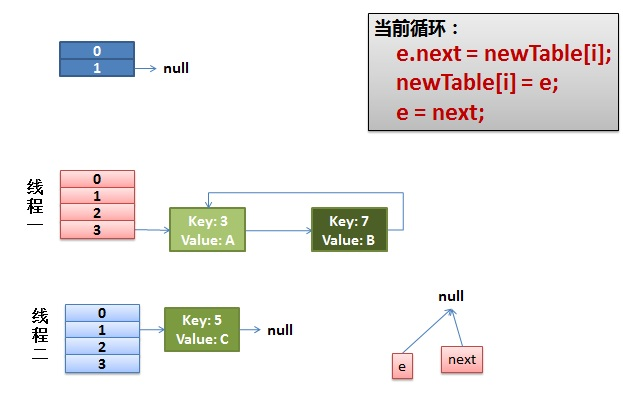## 三种解决方案

### Hashtable替换HashMap

Hashtable 是同步的，但由迭代器返回的 Iterator 和由所有 Hashtable 的“collection 视图方法”返回的 Collection 的 listIterator 方法都是快速失败的：在创建 Iterator 之后，如果从结构上对 Hashtable 进行修改，除非通过 Iterator 自身的移除或添加方法，否则在任何时间以任何方式对其进行修改，Iterator 都将抛出 ConcurrentModificationException。因此，面对并发的修改，Iterator 很快就会完全失败，而不冒在将来某个不确定的时间发生任意不确定行为的风险。由 Hashtable 的键和值方法返回的 Enumeration 不是快速失败的。

### Collections.synchronizedMap将HashMap包装起来

Map m = Collections.synchronizedMap(new HashMap());
...
Set s = m.keySet();  // Needn't be in synchronized block
...
synchronized(m) {  // Synchronizing on m, not s!
Iterator i = s.iterator(); // Must be in synchronized block
while (i.hasNext())
foo(i.next());
}


### ConcurrentHashMap替换HashMapposted @ 2016-04-18 01:01  Andrew.Zhou  阅读(55048)  评论(4编辑  收藏  举报